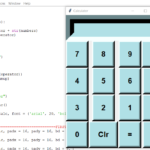## Calculator With Tkinter GUI In Python With Source Code

The Calculator With Tkinter GUI In Python is a simple project developed using Python. The project contains the numbers, operators, and signs like on a normal calculator. Here, the user can just click on the numbers they want in the calculations. Hence, th...

Type : Project

File Size : 1.0 KB# Similar Projects and Reports

#### Calculator With Tkinter GUI In Python With Source Code

The Calculator With Tkinter GUI In Python is a simple project developed using Python. The project contains the numbers, operators, and signs like on a normal calculator. Here, the user can just click on the numbers they want in the calculations. Hence, th...

#### Calculator With GUI In Python With Source Code

The Calculator With GUI In Python is a simple project developed using Python. The project contains the numbers, operators, and signs like in a normal calculator. So, the user cannot enter the number themselves, they can just click on the numbers they want...

#### Python Calculator In GUI With Source Code

The Python Calculator In GUI is a simple project developed using Python. The project contains the numbers, operators, and signs like on a normal calculator. Here, the user can just click on the numbers they want in the calculations. Hence, the user can us...

#### GUI CALCULATOR IN PYTHON WITH SOURCE CODE

The GUI Calculator In Python is a simple project developed using Python. The project contains the numbers, operators, and sign like in normal calculator. So, the user cannot enter the number themselves, they can just click on the numbers they want in the ...

#### CALCULATOR IN PYTHON WITH SOURCE CODE

This Calculator project In Python is a simple project developed using Python. The project contains the numbers, operators, and sign like in normal calculator. So, the user can enter any number they want in the calculations. Hence, the user can use a simpl...

#### Tip Calculator In Python with Source Code

The Tip Calculator In Python is a simple project developed using Python. The project is for the user convenient, for calculating the tip. This project is an interesting project. The user can calculate the tip amount that they can get from a single bill pa...